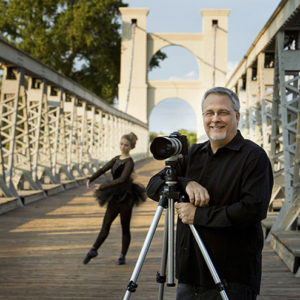#Calculating Exposure by Steve Kozak M. Photog., CR. PPA Certified

The basics of photography begin with correctly exposing the sensor by finding the right combination of f-stop and shutter speed. Exposure can be expressed in this formula:

Exposure = INTENSITY X TIME
or, E = IT

“Intensity” is the f-stop and “Time” is the shutter speed.

If the formula is true (which it is), then we can plug-in some numbers just to see what happens. So let’s do it…

E = I x T
E = F8 @ 1/125

(F8 and 1/125 are variables that I selected entirely at random for this example.)

“E” will represent the amount of light that reaches the sensor. If you remember any algebra, because there is an = in the equation, I can change my variables, so long as I keep them an equal value.

For Example:

E = 2×10, and E=4×5
(E is the same answer in both, but we used different variables.)

In my sample, I stated E = F8 @1/125. If for some reason I wanted to change the F8 to F5.6, could I do it? YES! As long as I keep the amount of light that reaches the film equal!

-If I move from F8 to F5.6, I am making the lens open up to let twice the amount of light reach the film.
-In order to remain equal, I have to cut the light in half by moving the shutter speed to the next faster speed.

E = F8 @ 125
And
E = F5.6 @ 250

F5.6 @ 1/250 would be an equal, or equivalent exposure to F8 @ 1/125 because in both cases, the exact amount of light reaches the film. As a matter of fact, there can be many equivalent exposures:

F22 @ 1/15
F16 @ 1/30
F11 @ 1/60
F8 @ 1/125
F5.6 @ 1/250
F4 @ 1/500
F2.8 @ 1/1000

Each of the above exposures yield the exact amount of light onto the film. This process is the same no matter which f-stop and shutter speed combination you choose to start out with. The hard part about exposure is figuring out which f-stop and shutter speed to start with in the first place. (We will cover this in-depth later.)

It is at this point that you may be thinking, “Gosh, If F8 @ 1/125 will work, why the heck would I want to change to F4 @ 1/500?” This is where we first begin to realize the magic of photography.

Remember, F-stops control depth of field, so changing from F8@1/125 to F4@1/500 allows you to create an image with a shallower depth of field.

This quiz is for logged in users only.